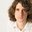Question-and-Answer Resource for the Building Energy Modeling Community
Get started with the Help page

# Fan Heat Gain CalculationI'm looking into the fan heat gain equations in the engineering reference guide, but I don't understand the logic. There are also a lot of missing equation references and things, so maybe the section isn't finished?

Specifically on pg 1051 (V8.8) at the end of section 17.4.2.4 says that fan heat loss to the air: Qtoair = Hfan +(Hmotor - Hfan) * fmotortoair, which I don't understand. H is defined as power [W], so this says that in a condition where fmotortoair = 0, the heat to the air is equal to the total power to the fan (mechanical power to the impeller I assume), which is obviously not true because a large portion of that power is converted to rotational energy. Then if fmotortoair > 0, some of that impeller power is subtracted for some reason from the total.

Any insights into what's going on would be appreciated, because right now this seems incorrect to me.

edit retag close merge delete

Sort by » oldest newest most votedIf a large portion of the mechanical power of the impeller would be converted to rotational energy, the the fan would constantly accelerate. In reality what happens, if you feed a fan's motor with constant power, then the fan starts to accelerate, and after a while it rotates with a constant speed. As soon as the fan is at constant speed, the power fed in to increase the rotational energy of the fan is equal to the power, that the fan feeds into the kinetic energy of the air. These two powers (motor-> fan & fan-> air) are in balance, that is why the fan rotates at constant speed, and why all this energy goes to heat (the air stops moving after a while and its energy dissipates to heat).
To me the equation looks correct. The minor things, that you may argue with, may be:

• Where exactly the kinetic energy of the air turns into heat. Now this calculation assumes, that this happens at the fan or in the duct, however some of the dissipation of kinetic energy of air to heat happens in the room, where the air stops moving due to friction. I think this is a very reasonable simplification.
• Other thing to nit-pick: With the extract fan, you throw away some of the kinetic energy of the air that is exiting the building at the air exhaust. So the system is not completely closed, a bit of energy is lost this way, which now is assumed to be heating the exhaust air.
more

@adambgnr that's a good point that it simplifies the fan kinetic energy to heat right at the fan, and I agree it's a reasonable simplification. I'm still not sure I follow any of the cases then the motor heat fraction is <0 though. As I understand it, that's just a proxy for how much of the motor is in the air stream, right? Why is Hfan subtracted from it then?

I think it is subtracted for f != 0, because the motor does two things with the electric energy it consumes: 1) dissipates it into heat right at the motor, because its efficiency is not 100% 2) gives the rest of the energy to the fan (via the belt) as mech. energy and then the fan dissipates it to heat. So this means, that the generated heat by the friction of the motor and the belt can be expressed as the power difference of the motor and the fan: Hmot - Hfan. this is 1). And case 2) is added to the energy of the air with Hfan.